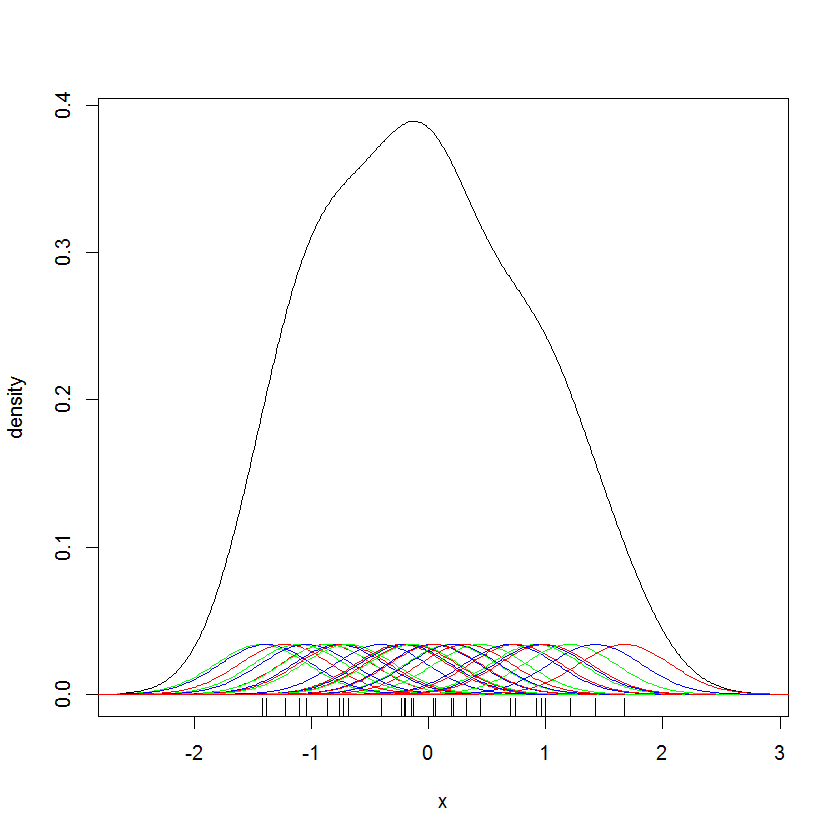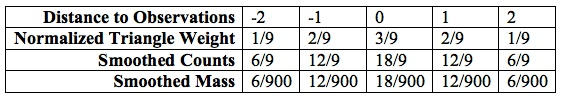Data Science Design Pattern #2: Variable-Width Kernel Smoothing

#Data Science Design Pattern #2:Variable-Width Kernel Smoothing

posted by Mosaic Data Science

A fundamental problem in applied statistics is estimating a probability mass function (PMF) or probability density function (PDF) from a set of independent, identically distributed observations.  When one is reasonably confident that a PMF or PDF belongs to a family of distributions having closed form, one can estimate the form’s parameters using frequentist techniques such as maximum likelihood estimation, or Bayesian techniques such as acceptance-rejection sampling.  In some cases however one would like not to assume a functional form, and instead “let the data speak for [itself].”[i]  We then work with an empirical PMF and the corresponding empirical cumulative distribution function (CDF).

Strictly speaking there is only one empirical CDF, which is a step function that jumps at each of our sample’s n observations.  Likewise, there is only one empirical PMF.  However, the empirical PMF may have either of two properties that make it inappropriate to use as a PMF in model building.

1. The empirical PMF samples a distribution having a smooth PDF, though not one from any of the usual closed-form distributions; and the goal is to estimate the smooth PDF.
1. The goal is to estimate a PMF; but any useful binning[ii] of the sample space will result in many or most bins containing zero mass, even though a sufficiently large sample would place positive mass in many or most bins.

In both cases, we need to smooth the empirical PMF into an estimated PMF or PDF by spreading the empirical PMF’s mass while preserving the shape information implicit in the empirical PMF.  That is, the value of an estimated PMF or PDF at a given point must derive from the empirical PMF’s mass at nearby points.[iii]

## Description

Kernel smoothing.  Kernel smoothing is the most popular nonparametric approach to constructing an estimated PMF or PDF.  It generalizes the idea of a moving average.  In technical terms, a kernel smoother redistributes mass around an observation according to two inputs:  a kernel function and a bandwidth.

A kernel function is a symmetrical PDF.  A typical kernel locates most of its mass near its center, with its mass declining rapidly with distance from the center.[iv]  The kernel’s shape dictates how much of an observation’s mass is spread at a given distance from the observation.

A bandwidth h is the maximum distance from the kernel’s center at which mass is spread.  Bandwidth is therefore integral in the discrete case.[v]  One scales the kernel function so that most or all of its mass falls within the bandwidth.  For example, a Gaussian kernel might be scaled so that its third standard deviation falls just within the bandwidth.

Traditionally bandwidth is constant.  Here we’re interested in allowing the bandwidth to vary.  We describe possible variable-bandwidth rules under “How it Works” below.

Continuous (PDF) smoother.  In the continuous case one locates a kernel at each observation, and mixes the kernels (with mixture weights of 1/n for each kernel) to obtain the estimated PDF.  For example, Figure 1 represents a Gaussian smoothing of 30 unit-normal random samples using the default bandwidth-selection rule of R’s density function, which results in a kernel having standard deviation of 0.3931.  The kernels around the sample (in red, green, and blue) are scaled by the mixture weight of 1/30.[vi]Figure 1:  Example Gaussian Smoothing

Here is the R code that generates Figure 1:

set.seed(124)
normalSample <- sort(rnorm(30))
normalSampleDensity <- density(x = normalSample, kernel = “gaussian”)
x = seq(-4, 4, length = 500)
plot(
normalSampleDensity,
xlab = ‘x’,
ylab = ‘density’,
main = ”
)
rug(normalSample)
colors <- c(“red”, “green”, “blue”)
for (i in 1:30){
y = dnorm(x, mean = normalSample[i], sd = normalSampleDensity\$bw) / 30
lines(x, y, col=colors[(i%%3)+1])
}

Discrete (PMF) smoother.  In the discrete case, one computes a set of kernel weights based on the kernel function and bandwidth, normalizing the weights so they sum to unity.  Then, a bin’s observation count is redistributed to that bin and the surrounding bins, weighted by the kernel weights.  Finally, all bins’ redistributed counts are normalized.

For example, suppose a study requiring an estimated PMF has n = 100 observations.  Table 1 illustrates a one-dimensional triangle smoothing of a bin containing six observations for h = 3 (so that mass is spread among the observation’s bin and the two surrounding bins on either side), assuming that the five bins do not receive mass from any other observations.Table 1:  Example Triangle-Smoothed PMF

Bandwidth selection.  The hardest part of implementing kernel smoothing, especially in the fixed-bandwidth case, is choosing an optimal bandwidth.  When the bandwidth is too narrow, the estimated PDF is too rough; when the bandwidth is too wide, the estimated PDF is too smooth.[vii]  Several approaches to fixed-bandwidth selection exist, and a fair treatment of the problem is beyond the scope of this post.[viii]  Formal optimization criteria are not always persuasive, despite their analytical virtues.  Visual intuition remains important.[ix]  See “How it Works” below for the variable-bandwidth case.

Choice of kernel.  The Epanechnikov kernel is optimal in the sense that it minimizes asymptotic mean integrated squared error.  Kernels’ efficiency is often compared to that of the Epanechnikov kernel.  Most common kernels’ efficiency is better than 90%, so the literature commonly asserts that kernel choice has only a weak effect on smoothing.[x]  Kernels are often chosen for their analytical properties instead.  When one requires an estimated PDF, Gaussian (normal) kernels are especially common.

Boundary values.  The other hard part of kernel smoothing is deciding how to smooth near the boundaries of the sample space.  Solutions tend to be domain specific.  For example, when time of year is one of the dimensions, smoothing observations near the beginning or end of the year requires wrapping the year’s endpoints together, so that smoothed mass can cross from e.g. December into January.  When dimensions are geospatial, one can smooth beyond the boundary of a land mass or property, truncate the result at the boundary, and then normalize.

## How it Works

Variable-width smoothing.  A variable-width smoother allows the bandwidth to vary so that mass spreads appropriately where the empirical PMF’s sparseness varies widely over the sample space.  Where mass is relatively dense, less smoothing occurs (the bandwidth is narrowed).  Where mass is sparse, more smoothing occurs.

Several rules are possible for varying bandwidth.[xi]  Some are expressed in terms of an observation’s location.  For example, the sample-point estimator varies bandwidth in proportion to the empirical density at the sample point.  Other rules are expressed in terms of the estimation location.  For example, the nearest-neighbor smoother assigns to a given estimation location the (normalized) linear combination of the k samples nearest to the bin, for some constant positive integer k, using kernel weights in the linear combination.  One bandwidth-selection heuristic for the nearest-neighbor smoother is k ≈ √n, but more modern heuristics exist; consult the references for details.  A maximum bandwidth may also be imposed, to limit smoothing in a manner appropriate to the domain.

## When to Use It

Kernel smoothing can reveal and preserve structural features of a PMF or PDF that a parametric estimate would obscure.  When the empirical PMF has highly variable density, use variable-width smoothing.  Variable-bandwidth kernel smoothing is more valuable in multiple dimensions, and can provide a good estimated PMF for Monte Carlo simulation.

## Example

Examples of variable-width smoothing abound in the scientific literature.  A good example is Kerry Emanuel et/al, “A Statistical Deterministic Approach to Hurricane Risk Assessment,” Bulletin of the American Meteorological Society, 87 (2006), pp. 299–314.[xii]  This research uses a smoothed PMF as the basis for a simulation study of hurricane risk.  The sample space of hurricane-origination locations contains sets of triples of the form (latitude, longitude, time).  The authors discretize the sample space to 0.5 degrees latitude and longitude, and five-day time periods.  The empirical PMF counts hurricane events since 1970, using the HURDAT database.  The study uses nearest-neighbor smoothing, with a limit of 15 days and 15 degrees.

Another interesting example is Maxim V. Shapovalov and Roland L. Dunbrack, Jr., “A Smoothed Backbone-Dependent Rotamer Library for Proteins Derived from Adaptive Kernel Density Estimates and Regressions,” Structure 19, pp. 844–858 (June 8, 2011).[xiii]  This study uses variable-width kernel smoothing for protein-structure determination, prediction, and design.

[i] Wolfgang Hardle, Smoothing Techniques With Implementation in S.  Springer Verlag (1991), p.4.

[ii] One way to make sure a binning is adequately fine grained is to experiment with different anchor points, to make sure the pattern revealed by the binning does not vary significantly with the choice of anchor point.  The first example on http://en.wikipedia.org/wiki/Multivariate_kernel_density_estimation (visited February 17, 2014) illustrates the point.

[iii] Many approaches to the problem exist.  See J.O. Ramsay and B.W. Silverman, Functional Data Analysis, Springer Verlag (1997).

[iv] Some common kernels are uniform, triangle, quadratic, quartic, Gaussian, and Epanechnikov.  See http://en.wikipedia.org/wiki/Kernel_(statistics)#Kernel_functions_in_common_use for a list with PDF graphs.

[v] Kernel smoothing is useful in several dimensions, and technically a different bandwidth—or even a different kernel function—could be used for each dimension.  When different dimensions use different units, and a single bandwidth and kernel are desired, one can standardize each dimension before smoothing.  The literature generally limits the number of dimensions to three.  Beyond this, combinatorial explosion in the number of bins means effective smoothing requires more raw data than is typically available.

[vi] The bandwidth-selection rule is taken from B.W. Silverman, Density Estimation for Statistics and Data Analysis, Chapman & Hall (1986).

[vii] See the graphical examples of under- and over-smoothing at http://ned.ipac.caltech.edu/level5/March02/Silverman/Silver2_4.html (visited February 18, 2014).

[viii] Start with the Wikipedia articles at  http://en.wikipedia.org/wiki/Kernel_density_estimation#Bandwidth_selection  and  http://en.wikipedia.org/wiki/Multivariate_kernel_density_estimation#Optimal_bandwidth_matrix_selection (visited February 18, 2014), and their references.  See Chapter 4 “Bandwidth Selection in Practice” of Hardle, ibid, for an extended practical treatment.  See also Chapter 4 “Bandwidth Selection” in M. P. Wand and M. C. Jones, Kernel Smoothing, Chapman & Hall (1995).

[ix] J.S. Marron and A.B. Tsybakov, “Visual Error Criteria for Qualitative Smoothing,” Journal of the American Statistical Association Vol. 90, No. 430 (June 1995), pp. 499-507, http://www.jstor.org/stable/2291060 (visited February 18, 2004).

[x] http://compdiag.molgen.mpg.de/docs/talk_05_01_04_stefanie.pdf (visited February 18, 2014).

[xi] For the technicalities, see Silverman, ibid; Stephan R. Stain, “Multivariate Locally Adaptive Density Estimation,” Computational Statistics & Data Analysis 39 (2002), pp. 165–186, http://private.igf.edu.pl/~jnn/Literatura_tematu/Sain_2002.pdf (visited February 18, 2014); and Wojciech Jarosz, Appendix C “Density Estimation,” Efficient Monte Carlo Methods for Light Transport in Scattering Media, PhD Dissertation U.C. San Diego, http://zurich.disneyresearch.com/~wjarosz/publications/dissertation/appendixC.pdf (visited February 17, 2014).

[xii] ftp://texmex.mit.edu/pub/emanuel/PAPERS/hurr_risk.pdf (visited February 18, 2004).  See also the supplement at ftp://texmex.mit.edu/pub/emanuel/PAPERS/hurr_risk.pdf (visited February 18, 2004).

[xiii] http://dx.doi.org/10.1016/j.str.2011.03.019 (visited February 18, 2004).

This site uses Akismet to reduce spam. Learn how your comment data is processed.